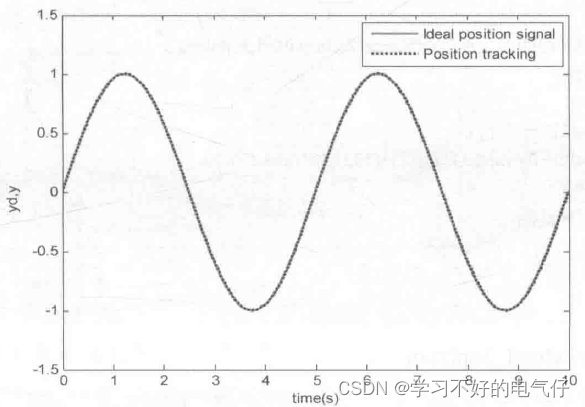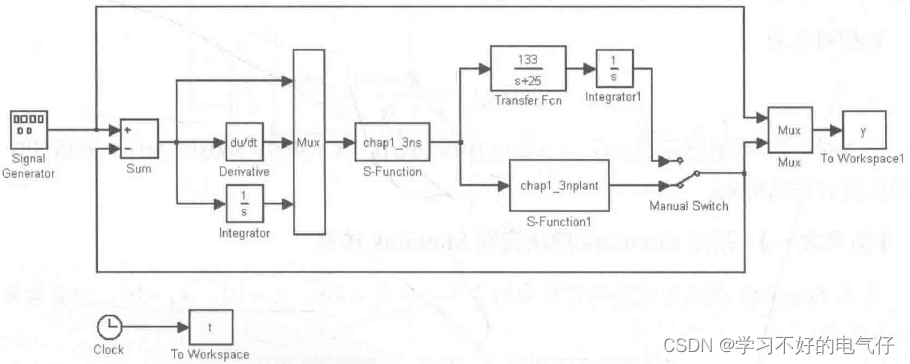news/2023/6/8 0:23:41function [sys,x0]=s_function(t,x,u,flag)

kp=60;

ki=1;

kd=3;

if flag==0

sys=[0,0.1,3,0,1]; %Outputs=1,Inputs=3,DirFeedthrough=0;

x0=[ ];

clseif flag==3

sys(1)=kp*u(1)+ki*u(2)+kd*u(3);

else

sys=[];

end

function [sys,x0]=s_function(t,x,u,flag)

if flag==0

sys=[2,0,1,1,0,0]; %ContStates=2,Outputs=1,Inputs=l

x0=[0.0];

elseif flag==1

sys(1)=x(2)

sys(2)=-(25+10*rands(1))*x(2)+(133+30*rands(1))*u;

elseif flag==3

sys(1)=x(1);

else

sys=[ ];

end

close all;

plot(t,y(:,1).'r',ty(:,2),'k:','linewidth' ,2);

xlabel( 'time(s)');ylabel('yd,y');

legend('ldeal position signal','Position tracking');

### 高等数学学习笔记——第六十八讲——隐函数存在定理

1. 问题引入——方程两边同时关于x求导数&#xff0c;求解隐函数导数 2. 要研究的问题&#xff1a;方程在什么条件下才能确定隐函数&#xff08;隐函数的存在性&#xff09;&#xff1b;隐函数的连续性、可微性、求导方法 3. 隐函数存在定理&#xff08;二元函数&#xff09; 4…